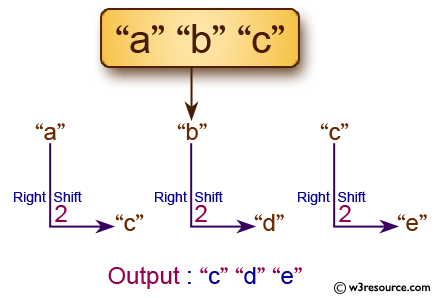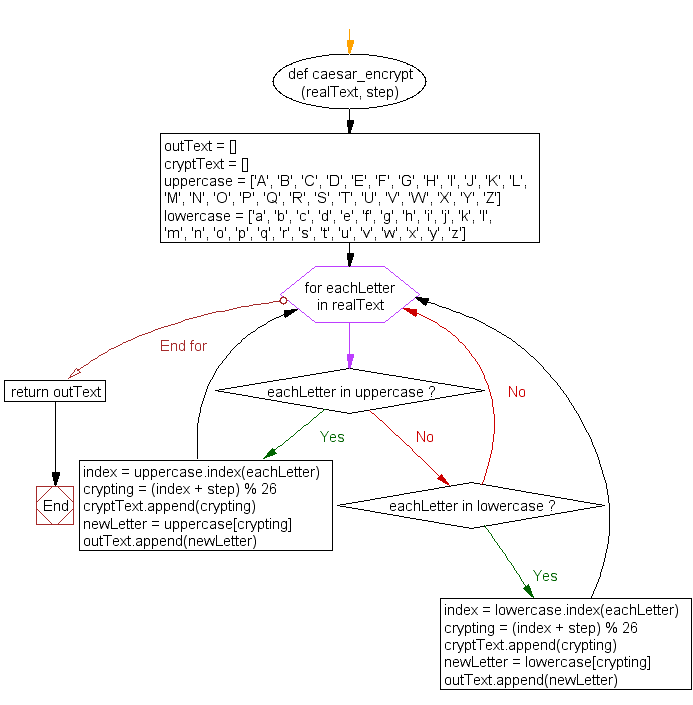﻿ Python: Create a Caesar encryption - w3resource

# Python: Create a Caesar encryption

## Python String: Exercise-25 with Solution

Write a Python program to create a Caesar encryption.

Note: In cryptography, a Caesar cipher, also known as Caesar's cipher, the shift cipher, Caesar's code or Caesar shift, is one of the simplest and most widely known encryption techniques. It is a type of substitution cipher in which each letter in the plaintext is replaced by a letter some fixed number of positions down the alphabet. For example, with a left shift of 3, D would be replaced by A, E would become B, and so on. The method is named after Julius Caesar, who used it in his private correspondence.Sample Solution:-

Python Code:

``````#https://gist.github.com/nchitalov/2f2b03e5cf1e19da1525
def caesar_encrypt(realText, step):
outText = []
cryptText = []

uppercase = ['A', 'B', 'C', 'D', 'E', 'F', 'G', 'H', 'I', 'J', 'K', 'L', 'M', 'N', 'O', 'P', 'Q', 'R', 'S', 'T', 'U', 'V', 'W', 'X', 'Y', 'Z']
lowercase = ['a', 'b', 'c', 'd', 'e', 'f', 'g', 'h', 'i', 'j', 'k', 'l', 'm', 'n', 'o', 'p', 'q', 'r', 's', 't', 'u', 'v', 'w', 'x', 'y', 'z']

for eachLetter in realText:
if eachLetter in uppercase:
index = uppercase.index(eachLetter)
crypting = (index + step) % 26
cryptText.append(crypting)
newLetter = uppercase[crypting]
outText.append(newLetter)
elif eachLetter in lowercase:
index = lowercase.index(eachLetter)
crypting = (index + step) % 26
cryptText.append(crypting)
newLetter = lowercase[crypting]
outText.append(newLetter)
return outText

code = caesar_encrypt('abc', 2)
print()
print(code)
print()
```
```

Sample Output:

```['c', 'd', 'e']
```

Flowchart:## Visualize Python code execution:

The following tool visualize what the computer is doing step-by-step as it executes the said program:

Python Code Editor:

Have another way to solve this solution? Contribute your code (and comments) through Disqus.

What is the difficulty level of this exercise?

Test your Programming skills with w3resource's quiz.

﻿

## Python: Tips of the Day

Sorting a list of lists:

Consider we have a list of lists:

```lst = [[3, 5], [6, 8], [4, 6], [5, 8], [6, 7], [5, 8]]
```

We can sort the list based on the first or second items of the inner lists by using the sort function with a lambda function.

```lst.sort(key = lambda inner:inner)
print(lst)
```

Output:

```[[3, 5], [4, 6], [6, 7], [6, 8], [5, 8], [5, 8]]
```

The list is sorted based on the second items. We can do the same with the first items just by changing the 1 to 0.

```lst.sort(key = lambda inner:inner)
print(lst)
```

Output:

`[[3, 5], [4, 6], [5, 8], [5, 8], [6, 8], [6, 7]]`